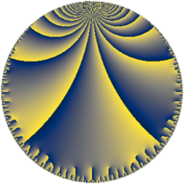Properties

 Label 37.8.fLevel $37$ Weight $8$ Character orbit 37.f Rep. character $\chi_{37}(7,\cdot)$ Character field $\Q(\zeta_{9})$ Dimension $132$ Newform subspaces $1$ Sturm bound $25$ Trace bound $0$

Related objects

Defining parameters

 Level: $$N$$ $$=$$ $$37$$ Weight: $$k$$ $$=$$ $$8$$ Character orbit: $$[\chi]$$ $$=$$ 37.f (of order $$9$$ and degree $$6$$) Character conductor: $$\operatorname{cond}(\chi)$$ $$=$$ $$37$$ Character field: $$\Q(\zeta_{9})$$ Newform subspaces: $$1$$ Sturm bound: $$25$$ Trace bound: $$0$$

Dimensions

The following table gives the dimensions of various subspaces of $$M_{8}(37, [\chi])$$.

Total New Old
Modular forms 144 144 0
Cusp forms 132 132 0
Eisenstein series 12 12 0

Trace form

 $$132 q + 15 q^{2} + 33 q^{3} - 279 q^{4} + 576 q^{5} - 12 q^{6} - 597 q^{7} - 3750 q^{8} - 3243 q^{9} + O(q^{10})$$ $$132 q + 15 q^{2} + 33 q^{3} - 279 q^{4} + 576 q^{5} - 12 q^{6} - 597 q^{7} - 3750 q^{8} - 3243 q^{9} - 6003 q^{10} - 15975 q^{11} - 4635 q^{12} - 15981 q^{13} - 13491 q^{14} + 38493 q^{15} - 74151 q^{16} + 37698 q^{17} - 20100 q^{18} - 66102 q^{19} - 176184 q^{20} - 142530 q^{21} + 132783 q^{22} + 140631 q^{23} - 38793 q^{24} - 544092 q^{25} - 423147 q^{26} + 130557 q^{27} + 603813 q^{28} + 22890 q^{29} - 319860 q^{30} - 1288044 q^{31} - 217866 q^{32} + 112344 q^{33} + 812019 q^{34} + 1479252 q^{35} + 8118132 q^{36} - 1664550 q^{37} + 305010 q^{38} - 1580340 q^{39} - 4681425 q^{40} - 2956593 q^{41} - 3553029 q^{42} - 1023528 q^{43} + 6409524 q^{44} + 1803336 q^{45} + 10219434 q^{46} - 2607204 q^{47} - 2984043 q^{48} - 6819531 q^{49} + 5724015 q^{50} - 1675833 q^{51} + 11768451 q^{52} + 2280177 q^{53} - 7803897 q^{54} - 3542196 q^{55} + 12168843 q^{56} - 85203 q^{57} - 12425487 q^{58} - 7014600 q^{59} + 2356788 q^{60} + 10840332 q^{61} + 6334368 q^{62} - 3331359 q^{63} - 26175624 q^{64} - 573195 q^{65} - 36720249 q^{66} + 6642918 q^{67} - 25277742 q^{68} - 1236438 q^{69} - 27401982 q^{70} + 20311041 q^{71} + 40093005 q^{72} + 38643780 q^{73} - 9531 q^{74} + 16639446 q^{75} + 5787405 q^{76} - 316971 q^{77} + 9363381 q^{78} + 17712534 q^{79} - 74410128 q^{80} - 10355109 q^{81} - 16702371 q^{82} - 31021176 q^{83} - 61626192 q^{84} + 23576445 q^{85} + 18579072 q^{86} + 104996631 q^{87} + 57279702 q^{88} - 13471320 q^{89} + 56306910 q^{90} - 2335911 q^{91} - 178133436 q^{92} + 24366258 q^{93} + 77086020 q^{94} - 26254407 q^{95} + 152523921 q^{96} + 14408415 q^{97} - 521205 q^{98} + 58375668 q^{99} + O(q^{100})$$

Decomposition of $$S_{8}^{\mathrm{new}}(37, [\chi])$$ into newform subspaces

Label Dim. $$A$$ Field CM Traces $q$-expansion
$a_{2}$ $a_{3}$ $a_{5}$ $a_{7}$
37.8.f.a $132$ $11.558$ None $$15$$ $$33$$ $$576$$ $$-597$$